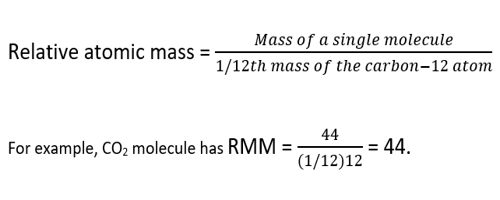Relative mass is an important concept in chemistry. It exists to simplify the process of working out the mass of an atom or molecule. Relative atomic mass (symbol: Ar) or atomic weight is a dimensionless physical quantity defined as the ratio of the average mass of atoms of a chemical element in a given sample to the atomic mass constant. These values tell you that a magnesium atom has twice the mass of a carbon atom and 24 times more mass than a hydrogen atom. They also tell you that hydrogen atoms have 12 times less mass than a carbon atom.

The relative atomic mass of an element is defined as the weight in grams of the number of atoms of the element contained in 12.00 g of carbon-12.

Atomic mass is expressed in terms of so-called atomic mass units (AMU). Where 1 amu = 1.661 x 10-24 g. This number is equal to one-twelfth of the mass of an atom of carbon-12. The atomic mass constant (symbol: mu) is defined as being 1/12 of the mass of a carbon-12 atom. Since both quantities in the ratio are masses, the resulting value is dimensionless; hence the value is said to be relative. All atomic masses are quoted relative to this value, and not surprisingly are referred to as the relative atomic mass of an element. To calculate the relative atomic mass of chlorine, the average mass of one atom of chlorine is found by considering 100 atoms of chlorine. 75.53 of these atoms each have a mass of 35 atomic mass units (AMU), and 24.47 atoms each have a mass of 37 AMU.Atoms contain protons, neutrons, and electrons. The electrons are arranged in shells around the nucleus. The periodic table is a chart of all the elements arranged in increasing atomic number. For a single given sample, the relative atomic mass of a given element is the weighted arithmetic mean of the masses of the individual atoms (including their isotopes) that are present in the sample. Atoms have such a small mass it is more convenient to know their masses compared to each other. Carbon is taken as the standard atom and has a relative atomic mass (Ar) of 12.

Atoms with an Ar that is more than this have a larger mass than a carbon atom. This quantity can vary substantially between samples because the sample’s origin (and therefore its radioactive history or diffusion history) may have produced unique combinations of isotopic abundances. The relative atomic mass of an element is a weighted average of the masses of the atoms of the isotopes – because if there is much more of one isotope then that will influence the average mass much more than the less abundant isotope will. For example, due to a different mixture of stable carbon-12 and carbon-13 isotopes, a sample of elemental carbon from volcanic methane will have a different relative atomic mass than one collected from plant or animal tissues.

Information Source: